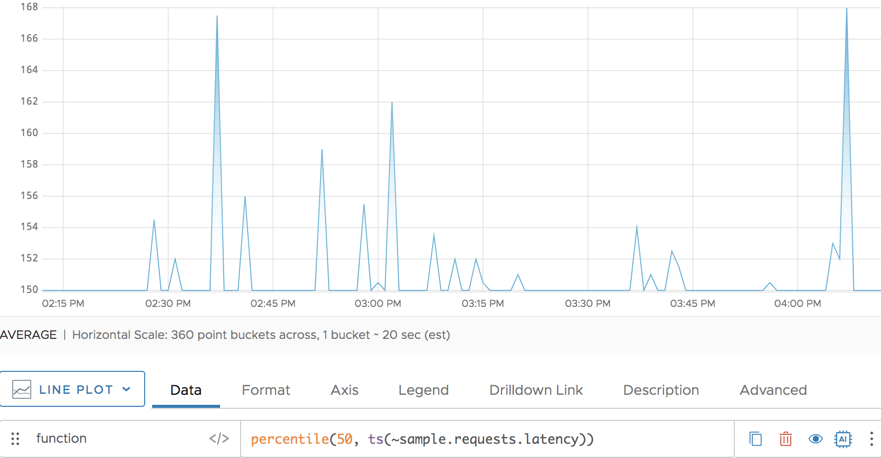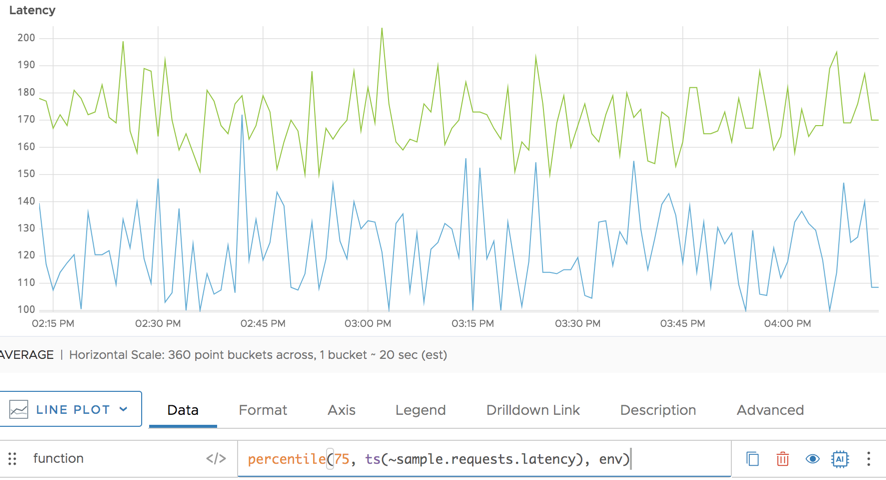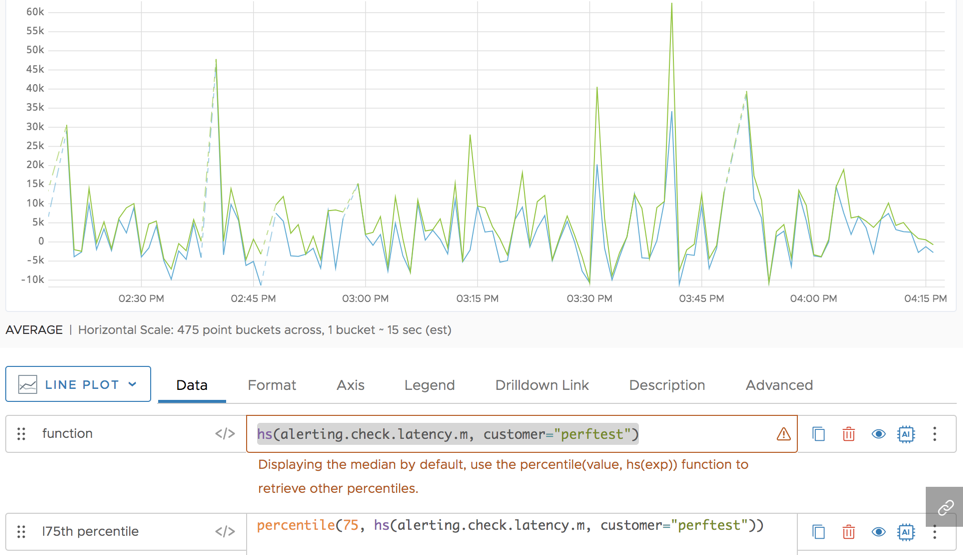Reference to the percentile() function

## Summary

``````percentile(<percentage>, <tsExpression>[, metrics|sources|sourceTags|pointTags|<pointTagKey>])

percentile(<percentage>, <hsExpression>)
``````

You can use `percentile()` with time series and with histograms.

 Time series aggregation function Returns the estimated `percentage` percentile across the set of time series described by `tsExpression`. The results might be computed from real reported values and interpolated values. Use `rawpercentile()` if you don’t want interpolation. Histogram conversion function Returns time series that consist of the `percentage` percentiles from each histogram distribution described by the `hsExpression`.

## Parameters

### Time-Series Aggregation Function

ParameterDescription
percentage A number greater than 0 and less than or equal to 100 that specifies the percentile of interest. You can include as many decimal places as you like.
tsExpression Expression describing the set of time series to estimate the specified percentile for.
metrics|sources|sourceTags|pointTags|<pointTagKey> Optional 'group by' parameter for organizing the time series into subgroups and then estimating the specified percentile for each subgroup. Use one or more parameters to group by metric names, source names, source tag names, point tag names, values for a particular point tag key, or any combination of these items. Specify point tag keys by name.

### Histogram Conversion Function

ParameterDescription
percentage A number from 0 to 100, inclusive, that specifies the percentile of interest. You can include as many decimal places as you like.
hsExpression Expression describing the histogram series to obtain the specified percentiles from.

## Description

You can use `percentile()`:

• With time series as an aggregation function.
• With histogram series as a conversion function.

### Time-Series Aggregation Function

The `percentile()` aggregation function lets you estimate the specified percentile for the data values at each moment in time, across the time series that are represented by the expression.

A percentile is a value below which a particular percentage of data values fall. You specify the percentage as a positive real number that is less than or equal to 100. For example, if you specify a percentage of 99.4, `percentile()` returns a value at the 99.4th percentile for each successive moment in time. You can specify a percentage of 50 to obtain the median value across the time series. See Computing an Estimated Percentile for more details.

By default, `percentile()` returns a single series of percentile values across all time series. You can optionally group the time series based on one or more characteristics, and obtain a separate series of percentile values for each group.

If any time series has data gaps, `percentile()` fills them in by interpolation whenever possible.

#### Grouping

Like all aggregation functions, `percentile()` returns a single series of percentiles by default. You can include a ‘group by’ parameter to return percentiles for separate groups of time series that share common metric names, source names, source tags, point tags, or values for a particular point tag key. The function returns a separate series of results corresponding to each group.

You can specify multiple ‘group by’ parameters to group the time series based on multiple characteristics. For example, `percentile(ts(50, "cpu.cpu*"), metrics, Customer)` first groups by metric names, and then groups by the values of the `Customer` point tag.

#### Interpolation

If any time series has gaps in its data, Wavefront attempts to fill these gaps with interpolated values before applying the function. A value can be interpolated into a time series only if at least one other time series reports a real data value at the same moment in time.

Within a given time series, an interpolated value is calculated from two real reported values on either side of it. Sometimes interpolation is not possible–for example, when a new value has not been reported yet in a live-view chart. In this case, Wavefront finds the last known reported value in the series, and assigns it to any subsequent moment in time for which a real reported data value is present in some other time series. We use the last known reported value only if interpolation can’t occur and if the last known reported value has been reported within the last 15% of the query time in the chart window.

You can use `rawpercentile()` to suppress interpolation. See Standard Versus Raw Aggregation Functions.

#### Computing an Estimated Percentile

A percentile is a value below which a particular percentage of data values fall. For example, in a given group of data values, the 75th percentile is a value that is greater than 75% of the data values in that group.

In general, percentiles can be estimated in various ways. The `percentile()` function computes the estimated percentile value for a given moment in time as follows:

1. Obtain the set of reported and/or interpolated data values, one from each time series in the group.
2. Sort these data values into an ordered list.
3. Use the specified `percentage` to compute the estimated percentile position in the list, and then:
• If the estimated percentile position is a whole number, return the data value at that position.
• If the estimated percentile position is fractional, find the two data values on either side of it, and use them to interpolate the value to return.
• If the estimated percentile position falls before the list’s first position (or after the list’s last position), return the smallest (or largest) data value in the list.

The formula for computing the estimated percentile position `pos` is `pos = percentage * (n+1)/100`, where `n` is the number of data values.

### Histogram Conversion Function

The `percentile()` histogram conversion function computes the specified percentile for the data values in each distribution of the histogram series that are represented by the expression. The percentiles for a given histogram series are returned as a separate time series that contains one data point corresponding to each input distribution.

A percentile is a value below which a particular percentage of data values fall. You specify the percentage as a real number that is less than or equal to 100. For example, if you specify a percentage of 99.4, `percentile()` returns a value at the 99.4th percentile from each successive distribution. You can specify a percentage of 50 to obtain the median value across the histogram series.

`percentile()` is a histogram conversion function because it takes histogram distributions as input, and returns time series. You can therefore use a histogram conversion function as a `tsExpression` parameter in a time series query function.

## Examples

### Time-Series Aggregation Function

The following simple example shows the median (50th percentile) for the requests latency. It’s a single line because `percentile()` is an aggregation function.The following example shows the 75th percentile for the request latency. Here, we group by the `env` point tag and get a line for `env=production` and a line for `env=dev`.### Histogram Conversion Function

In the following example, the green line shows the result of using `percentile()` to compute the 75th percentile values from the distributions described by `hsExpression`.`percentile()` returns a separate time series for each input histogram series. In this example, the `hsExpression` represents a single histogram series, so the result is a single time series. (In contrast, when `percentile()` is applied to a `tsExpression`, a single returned time series might be the result of combining multiple input time series.)